# Motion problem

From Levíc to Košíc go car at speed 81 km/h.
From Košíc to Levíc go another car at speed 69 km/h.

How many minutes before the meeting will be cars 27 km away?

Correct result:

t =  10.8 min

#### Solution:

$v_{1}=81 \ \text{km/h} \ \\ v_{2}=69 \ \text{km/h} \ \\ s=27 \ \text{km} \ \\ \ \\ v=v_{1}+v_{2}=81+69=150 \ \text{km/h} \ \\ t_{1}=\dfrac{ s }{ v }=\dfrac{ 27 }{ 150 }=\dfrac{ 9 }{ 50 }=0.18 \ \text{h} \ \\ t=t_{1} \rightarrow h=t_{1} \cdot \ 60 \ h=0.18 \cdot \ 60 \ h=10.8 \ h=\dfrac{ 54 }{ 5 }=10.8 \ \text{min}$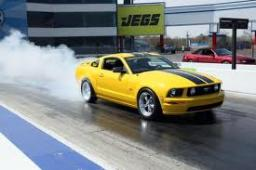Our examples were largely sent or created by pupils and students themselves. Therefore, we would be pleased if you could send us any errors you found, spelling mistakes, or rephasing the example. Thank you!

Leave us a comment of this math problem and its solution (i.e. if it is still somewhat unclear...):Be the first to comment!Tips to related online calculators
Need help calculate sum, simplify or multiply fractions? Try our fraction calculator.
Check out our ratio calculator.
Do you have a linear equation or system of equations and looking for its solution? Or do you have quadratic equation?
Do you want to convert length units?
Do you want to convert velocity (speed) units?
Do you want to convert time units like minutes to seconds?

## Next similar math problems:

• Three-digit numbersWe have digits 0,1,4,7 that cannot be repeated. How many three-digit numbers can we write from them? You can help by listing all the numbers.Adam has half the money in his right pocket than in his left pocket. If he transferred 40 crowns from the left pocket to the right, he would have the same in both pockets. Calculate how many crowns does Adam have in his left pocket more than in his right?
• Lookout towerHow high is the lookout tower? If each step was 3 cm lower, there would be 60 more of them on the lookout tower. If it was 3 cm higher again, it would be 40 less than it is now.
• Research in schoolFor particular research in high school, four pupils are to be selected from a class with 30 pupils. Calculate the number of all possible results of the select and further calculate the number of all possible results, if it depends on the order in which th
• Large familyThe average age of all family members (children, mother, father, grandmother, grandfather) is 29 years. The average age of parents is 40 years, grandparents 66 years and all children are 5 years. How many children are there in this family?
• Gold, silver, bronzeHow many ways can we divide gold, silver, bronze medails if there are 6 people competing?
• Six questions testThere are six questions in the test. There are 3 answers to each - only one is correct. In order for a student to take the exam, at least four questions must be answered correctly. Alan didn't learn at all, so he circled the answers only by guessing. What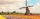How large must the group of people be so that the probability that two people have a birthday on the same day of the year is greater than 90%?
• The manufacturer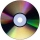The manufacturer found that 3% of the plates produced had a malfunction. Of the compliant, 75% are first and 25% second. What is the probability of producing first and second class plates?
• ProbabilityHow probable is a randomly selected three-digit number divisible by five or seven?
• The testThe test contains four questions, and there are five different answers to each of them, of which only one is correct, the others are incorrect. What is the probability that a student who does not know the answer to any question will guess the right answer
• Double probabilityThe probability of success of the planned action is 60%. What is the probability that success will be achieved at least once if this action is repeated twice?
• Tournament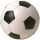How many matches will be played in a football tournament in which there are two groups of 5 teams if one match is played in groups with each other and the group winners play a match for the overall winner of the tournament?
• A farmer's heritage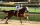A farmer died leaving his 17 horses to his 3 sons. When his sons opened up the Will it read: My eldest son should get 1/2 (half) of total horses; My middle son should be given 1/3rd (one-third) of the total horses; My youngest son should be given 1/9th (
• Columns of two and threeWhen students in one class stand in columns of two there is none left. When he stands in columns of three, there is one student left. There are 5 more double columns than three columns. How many students are in the class?
• Quiz or testI have a quiz with 20 questions. Each question has 4 multiple choice answers, A, B, C, D. THERE IS NO WAY TO KNOW THE CORRECT ANSWER OF ANY GIVEN QUESTION, but the answers are static, in that if the "correct" answer to #1 = C, then it will always be equal
• Five letters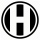How many ways can five letters be arranged?